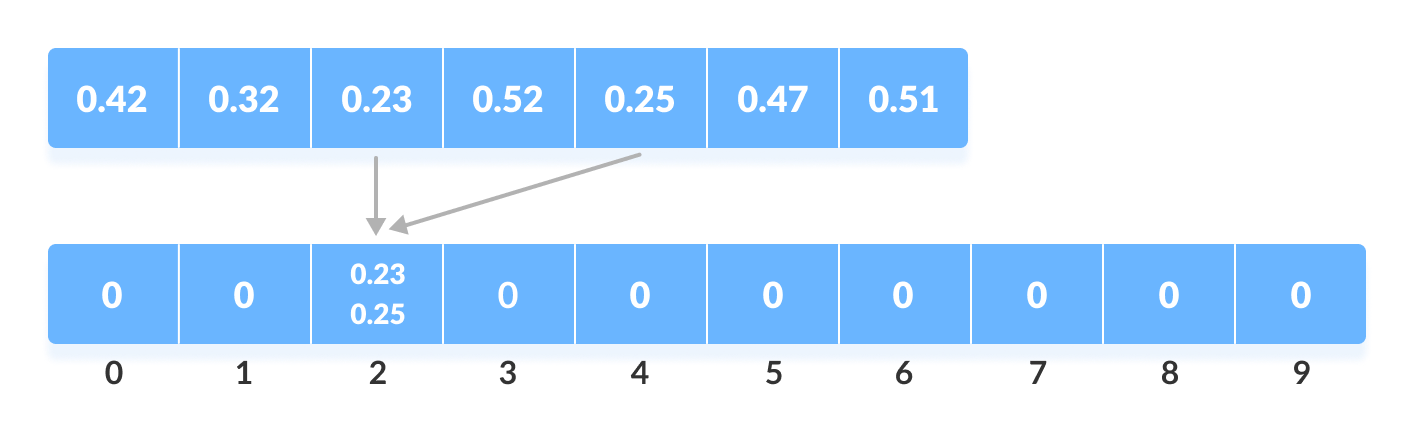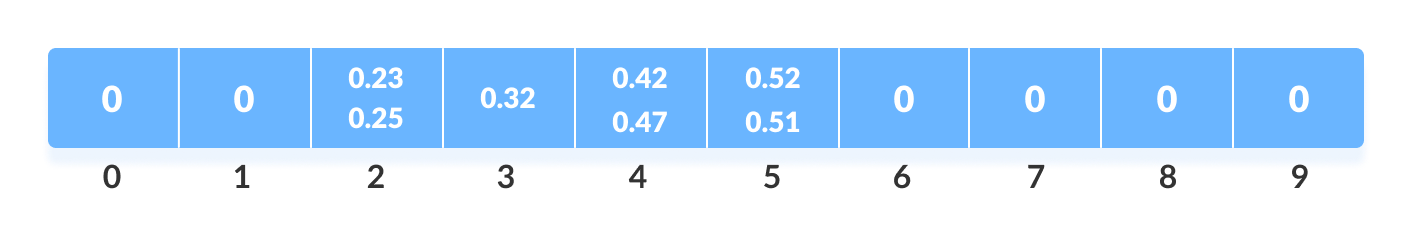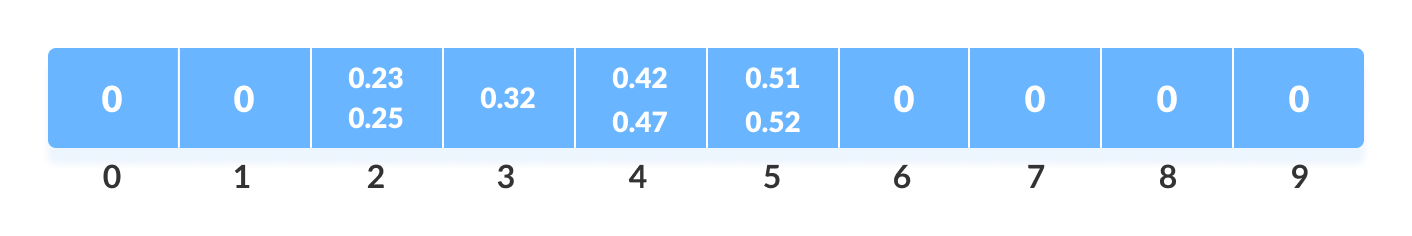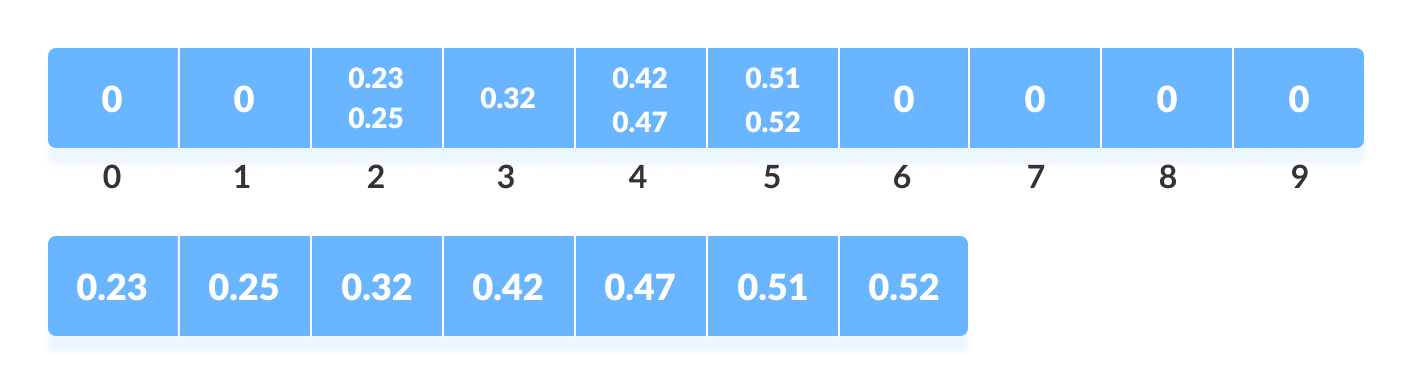# Bucket Sort Algorithm

In this tutorial, you will learn about the bucket sort algorithm and its implementation in Python, Java, C, and C++.

Bucket Sort is a sorting algorithm that divides the unsorted array elements into several groups called buckets. Each bucket is then sorted by using any of the suitable sorting algorithms or recursively applying the same bucket algorithm.

Finally, the sorted buckets are combined to form a final sorted array.

Scatter Gather Approach

The process of bucket sort can be understood as a scatter-gather approach. Here, elements are first scattered into buckets then the elements in each bucket are sorted. Finally, the elements are gathered in order.

## Working of Bucket Sort

Create an array of size 10. Each slot of this array is used as a bucket for storing elements.Array in which each position is a bucket
2. Insert elements into the buckets from the array. The elements are inserted according to the range of the bucket.

In our example code, we have buckets each of ranges from 0 to 1, 1 to 2, 2 to 3,...... (n-1) to n.
Suppose, an input element is `.23` is taken. It is multiplied by `size = 10` (ie. `.23*10=2.3`). Then, it is converted into an integer (ie. `2.3≈2`). Finally, .23 is inserted into bucket-2.Insert elements into the buckets from the array
Similarly, .25 is also inserted into the same bucket. Everytime, the floor value of the floating point number is taken.

If we take integer numbers as input, we have to divide it by the interval (10 here) to get the floor value.

Similarly, other elements are inserted into their respective buckets.Insert all the elements into the buckets from the array
3. The elements of each bucket are sorted using any of the stable sorting algorithms. Here, we have used quicksort (inbuilt function).Sort the elements in each bucket
4. The elements from each bucket are gathered.

It is done by iterating through the bucket and inserting an individual element into the original array in each cycle. The element from the bucket is erased once it is copied into the original array.Gather elements from each bucket

## Bucket Sort Algorithm

``````bucketSort()
create N buckets each of which can hold a range of values
for all the buckets
initialize each bucket with 0 values
for all the buckets
put elements into buckets matching the range
for all the buckets
sort elements in each bucket
gather elements from each bucket
end bucketSort``````

## Bucket Sort Code in Python, Java, and C/C++

``````# Bucket Sort in Python

def bucketSort(array):
bucket = []

# Create empty buckets
for i in range(len(array)):
bucket.append([])

# Insert elements into their respective buckets
for j in array:
index_b = int(10 * j)
bucket[index_b].append(j)

# Sort the elements of each bucket
for i in range(len(array)):
bucket[i] = sorted(bucket[i])

# Get the sorted elements
k = 0
for i in range(len(array)):
for j in range(len(bucket[i])):
array[k] = bucket[i][j]
k += 1
return array

array = [.42, .32, .33, .52, .37, .47, .51]
print("Sorted Array in descending order is")
print(bucketSort(array))``````
``````// Bucket sort in Java

import java.util.ArrayList;
import java.util.Collections;

public class BucketSort {
public void bucketSort(float[] arr, int n) {
if (n <= 0)
return;
@SuppressWarnings("unchecked")
ArrayList<Float>[] bucket = new ArrayList[n];

// Create empty buckets
for (int i = 0; i < n; i++)
bucket[i] = new ArrayList<Float>();

// Add elements into the buckets
for (int i = 0; i < n; i++) {
int bucketIndex = (int) arr[i] * n;
}

// Sort the elements of each bucket
for (int i = 0; i < n; i++) {
Collections.sort((bucket[i]));
}

// Get the sorted array
int index = 0;
for (int i = 0; i < n; i++) {
for (int j = 0, size = bucket[i].size(); j < size; j++) {
arr[index++] = bucket[i].get(j);
}
}
}

// Driver code
public static void main(String[] args) {
BucketSort b = new BucketSort();
float[] arr = { (float) 0.42, (float) 0.32, (float) 0.33, (float) 0.52, (float) 0.37, (float) 0.47,
(float) 0.51 };
b.bucketSort(arr, 7);

for (float i : arr)
System.out.print(i + "  ");
}
}``````
``````// Bucket sort in C

#include <stdio.h>
#include <stdlib.h>

#define NARRAY 7   // Array size
#define NBUCKET 6  // Number of buckets
#define INTERVAL 10  // Each bucket capacity

struct Node {
int data;
struct Node *next;
};

void BucketSort(int arr[]);
struct Node *InsertionSort(struct Node *list);
void print(int arr[]);
void printBuckets(struct Node *list);
int getBucketIndex(int value);

// Sorting function
void BucketSort(int arr[]) {
int i, j;
struct Node **buckets;

// Create buckets and allocate memory size
buckets = (struct Node **)malloc(sizeof(struct Node *) * NBUCKET);

// Initialize empty buckets
for (i = 0; i < NBUCKET; ++i) {
buckets[i] = NULL;
}

// Fill the buckets with respective elements
for (i = 0; i < NARRAY; ++i) {
struct Node *current;
int pos = getBucketIndex(arr[i]);
current = (struct Node *)malloc(sizeof(struct Node));
current->data = arr[i];
current->next = buckets[pos];
buckets[pos] = current;
}

// Print the buckets along with their elements
for (i = 0; i < NBUCKET; i++) {
printf("Bucket[%d]: ", i);
printBuckets(buckets[i]);
printf("\n");
}

// Sort the elements of each bucket
for (i = 0; i < NBUCKET; ++i) {
buckets[i] = InsertionSort(buckets[i]);
}

printf("-------------\n");
printf("Bucktets after sorting\n");
for (i = 0; i < NBUCKET; i++) {
printf("Bucket[%d]: ", i);
printBuckets(buckets[i]);
printf("\n");
}

// Put sorted elements on arr
for (j = 0, i = 0; i < NBUCKET; ++i) {
struct Node *node;
node = buckets[i];
while (node) {
arr[j++] = node->data;
node = node->next;
}
}

return;
}

// Function to sort the elements of each bucket
struct Node *InsertionSort(struct Node *list) {
struct Node *k, *nodeList;
if (list == 0 || list->next == 0) {
return list;
}

nodeList = list;
k = list->next;
nodeList->next = 0;
while (k != 0) {
struct Node *ptr;
if (nodeList->data > k->data) {
struct Node *tmp;
tmp = k;
k = k->next;
tmp->next = nodeList;
nodeList = tmp;
continue;
}

for (ptr = nodeList; ptr->next != 0; ptr = ptr->next) {
if (ptr->next->data > k->data)
break;
}

if (ptr->next != 0) {
struct Node *tmp;
tmp = k;
k = k->next;
tmp->next = ptr->next;
ptr->next = tmp;
continue;
} else {
ptr->next = k;
k = k->next;
ptr->next->next = 0;
continue;
}
}
return nodeList;
}

int getBucketIndex(int value) {
return value / INTERVAL;
}

void print(int ar[]) {
int i;
for (i = 0; i < NARRAY; ++i) {
printf("%d ", ar[i]);
}
printf("\n");
}

// Print buckets
void printBuckets(struct Node *list) {
struct Node *cur = list;
while (cur) {
printf("%d ", cur->data);
cur = cur->next;
}
}

// Driver code
int main(void) {
int array[NARRAY] = {42, 32, 33, 52, 37, 47, 51};

printf("Initial array: ");
print(array);
printf("-------------\n");

BucketSort(array);
printf("-------------\n");
printf("Sorted array: ");
print(array);
return 0;
}``````
``````// Bucket sort in C++

#include <iomanip>
#include <iostream>
using namespace std;

#define NARRAY 7   // Array size
#define NBUCKET 6  // Number of buckets
#define INTERVAL 10  // Each bucket capacity

struct Node {
int data;
struct Node *next;
};

void BucketSort(int arr[]);
struct Node *InsertionSort(struct Node *list);
void print(int arr[]);
void printBuckets(struct Node *list);
int getBucketIndex(int value);

// Sorting function
void BucketSort(int arr[]) {
int i, j;
struct Node **buckets;

// Create buckets and allocate memory size
buckets = (struct Node **)malloc(sizeof(struct Node *) * NBUCKET);

// Initialize empty buckets
for (i = 0; i < NBUCKET; ++i) {
buckets[i] = NULL;
}

// Fill the buckets with respective elements
for (i = 0; i < NARRAY; ++i) {
struct Node *current;
int pos = getBucketIndex(arr[i]);
current = (struct Node *)malloc(sizeof(struct Node));
current->data = arr[i];
current->next = buckets[pos];
buckets[pos] = current;
}

// Print the buckets along with their elements
for (i = 0; i < NBUCKET; i++) {
cout << "Bucket[" << i << "] : ";
printBuckets(buckets[i]);
cout << endl;
}

// Sort the elements of each bucket
for (i = 0; i < NBUCKET; ++i) {
buckets[i] = InsertionSort(buckets[i]);
}

cout << "-------------" << endl;
cout << "Bucktets after sorted" << endl;
for (i = 0; i < NBUCKET; i++) {
cout << "Bucket[" << i << "] : ";
printBuckets(buckets[i]);
cout << endl;
}

// Put sorted elements on arr
for (j = 0, i = 0; i < NBUCKET; ++i) {
struct Node *node;
node = buckets[i];
while (node) {
arr[j++] = node->data;
node = node->next;
}
}

for (i = 0; i < NBUCKET; ++i) {
struct Node *node;
node = buckets[i];
while (node) {
struct Node *tmp;
tmp = node;
node = node->next;
free(tmp);
}
}
free(buckets);
return;
}

// Function to sort the elements of each bucket
struct Node *InsertionSort(struct Node *list) {
struct Node *k, *nodeList;
if (list == 0 || list->next == 0) {
return list;
}

nodeList = list;
k = list->next;
nodeList->next = 0;
while (k != 0) {
struct Node *ptr;
if (nodeList->data > k->data) {
struct Node *tmp;
tmp = k;
k = k->next;
tmp->next = nodeList;
nodeList = tmp;
continue;
}

for (ptr = nodeList; ptr->next != 0; ptr = ptr->next) {
if (ptr->next->data > k->data)
break;
}

if (ptr->next != 0) {
struct Node *tmp;
tmp = k;
k = k->next;
tmp->next = ptr->next;
ptr->next = tmp;
continue;
} else {
ptr->next = k;
k = k->next;
ptr->next->next = 0;
continue;
}
}
return nodeList;
}

int getBucketIndex(int value) {
return value / INTERVAL;
}

// Print buckets
void print(int ar[]) {
int i;
for (i = 0; i < NARRAY; ++i) {
cout << setw(3) << ar[i];
}
cout << endl;
}

void printBuckets(struct Node *list) {
struct Node *cur = list;
while (cur) {
cout << setw(3) << cur->data;
cur = cur->next;
}
}

// Driver code
int main(void) {
int array[NARRAY] = {42, 32, 33, 52, 37, 47, 51};

cout << "Initial array: " << endl;
print(array);
cout << "-------------" << endl;

BucketSort(array);
cout << "-------------" << endl;
cout << "Sorted array: " << endl;
print(array);
}``````

## Bucket Sort Complexity

Time Complexity Space Complexity Best O(n+k) Worst O(n2) Average O(n) O(n+k) Yes

• Worst Case Complexity: `O(n2)`
When there are elements of close range in the array, they are likely to be placed in the same bucket. This may result in some buckets having more number of elements than others.
It makes the complexity depend on the sorting algorithm used to sort the elements of the bucket.
The complexity becomes even worse when the elements are in reverse order. If insertion sort is used to sort elements of the bucket, then the time complexity becomes `O(n2)`.
• Best Case Complexity: `O(n+k)`
It occurs when the elements are uniformly distributed in the buckets with a nearly equal number of elements in each bucket.
The complexity becomes even better if the elements inside the buckets are already sorted.
If insertion sort is used to sort elements of a bucket then the overall complexity in the best case will be linear ie. `O(n+k)`. `O(n)` is the complexity for making the buckets and `O(k)` is the complexity for sorting the elements of the bucket using algorithms having linear time complexity at the best case.
• Average Case Complexity: `O(n)`
It occurs when the elements are distributed randomly in the array. Even if the elements are not distributed uniformly, bucket sort runs in linear time. It holds true until the sum of the squares of the bucket sizes is linear in the total number of elements.

## Bucket Sort Applications

Bucket sort is used when:

• input is uniformly distributed over a range.
• there are floating point values